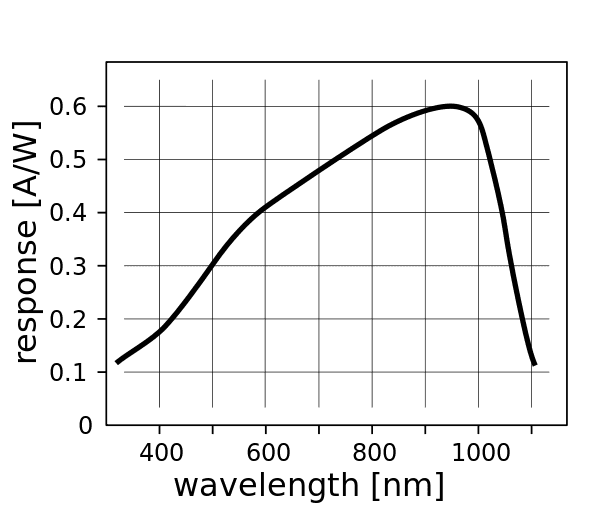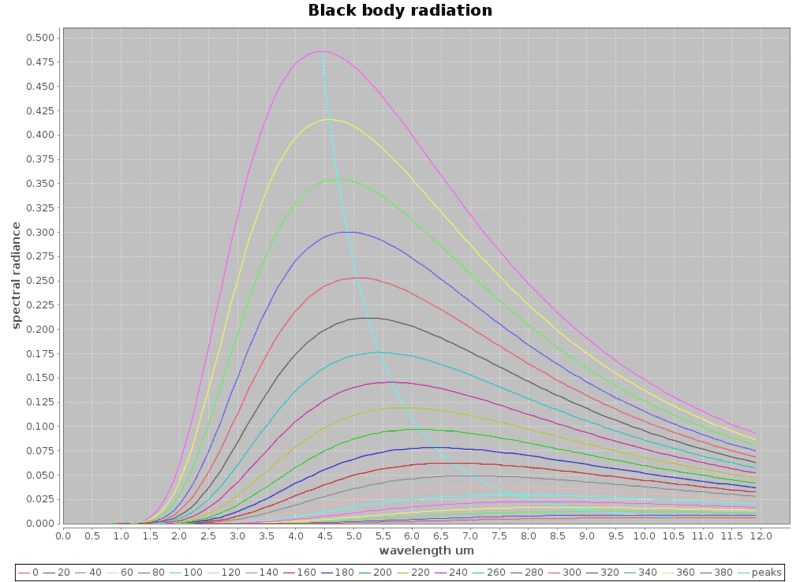# Infrared thermometry remote sensing

hi all,

This isn't simply physics but is very much related. i'm trying to make an infrared thermometer or some means of remote temperature measurements for temperatures from 0 degree C to around 400 degree C

I started with looking up low cost silicon photo diodes / photo transistors e.g. on ebay etc.
I noted that most of them have a peak sensitivity range around 950nm and spectral response that looks as follows
https://en.wikipedia.org/wiki/Photodiodehttps://en.wikipedia.org/wiki/Planck's_law
And to get a feel of what it would take: Using Planck's equation, I did a little program and plotted the black body radiation curves based on planck's equation for the black body radiation radiance against wavelengths for the various temperatures. The curves near the bottom start from 0 deg C to around 380 deg C for every 20 degree C rise in temperatures. I get these curves which are rather nice.Now I realised I've got a problem, silicon photo diodes can only detect well below 1.5um wavelengths which puts it outside the blackbody radiation ranges i'm trying to measure / detect

I started researching other means and run into thermopile detectors, e.g. ts-118
http://lusosat.org/hardware/200612916515751780.pdf
however, based on the spectral response, these thermopile detectors has an infrared filter that restricts radiation reaching the sensor to the range of 8-15 um, this is actually that around room temperatures or body temperatures (it is useful for a particular purpose)

The trouble is, if i use the thermopile sensor to measure the higher temperatures e.g. 300 deg C, the wavelengths narrowed significantly to around 4.5um, and a significant useful range of radiance energies could not be deployed for the measurements. While i'd think the thermopile detector would still be sufficiently sensitive to detect the higher temperatures, i'm limited to the sensitivity in the range of 8-15 um

I'm now wishing to use a different solution, similar to the nature of thermopile sensors which actually use the Seeback effect (https://en.wikipedia.org/wiki/Thermoelectric_effect#Seebeck_effect) to literally measure temperatures. i'd like to use some kind of temperature sensors e.g. a thermistor https://en.wikipedia.org/wiki/Thermistor
to measure the temperature at the probe. this temperature rise would be caused primarily by absorbing the black body radiation and my guess is it'd reach some equilibrium state with a stable temperature after a while.

the problem is how do i determine the temperature of the black body remotely from the temperature which i am able to measure at the thermistor/probe?
are there other (better) means to measure the temperature remotely (e.g. infrared etc)?
wikipedia has an article on pyrometers but is somewhat short on the physics / equations etc
https://en.wikipedia.org/wiki/Pyrometer

#### Attachments

Last edited:

anorlunda
Staff Emeritus
Try searching "photonic thermometry".

hilbert2
Gold Member
This kind of instruments have the problem of possible false results if the hot object has an emission spectrum very different from a blackbody, or if the medium between the sensor and the object is too effective in absorbing radiation at the control wavelengths that you're measuring to find the temperature.

thanks for the response ! :)
i decided to make an attempt at a thought experiment

making an attempted start from Stefan–Boltzmann law
based on the clues from wikipedia
https://en.wikipedia.org/wiki/Stefan–Boltzmann_law
https://en.wikipedia.org/wiki/Luminosity
$$j*= \sigma T^4 \\ where \space T \text { is temperature at source in K } \\ L= \sigma A T^4 \dots (1) \\ where \space \sigma = 5.670373 \times 10^{-8}\, \mathrm{W\, m^{-2}K^{-4}} \\ irradiance \space per \space unit \space area \space E = \varepsilon L / 4 \pi r^2 \\ where \space r \text { is distance between source and probe } \\ and \space \varepsilon \text { is emissivity } < 1 \\ \text {power received at probe} \space P = a \varepsilon L / 4 \pi r^2 \\ where \space a \text { is cross section area of the probe facing the source } \\ \text{ substituting (1) power received at probe} \space P = a \varepsilon \sigma A T^4 / 4 \pi r^2 \dots (2) \\ \text { if we aggregrate the items which are basically constant } \space P \propto T ^ 4 / r^2$$

i'm not too sure if the above is after all correct, but that if P (power received at probe) is
$$P \propto T ^ 4 / r^2 \\ where \space T \space \text {is temperature of source in K} \\ and \space r \space \text { is distance between source and probe}$$, it would seem quite possible to use a thermistor measure temperature at the probe) when temperature rises the energy would be conducted away or some emitted and reach some steady state temperature at the probe. this temperature could then be related to the source temperature remotely

if i make a further simplifying assumption that the heat/power received at the probe is simply conducted away, and using the heat conduction equations
https://en.wikipedia.org/wiki/Thermal_conduction#Integral_form
$$\big. \frac{Q}{\Delta t} = -k A \frac{\Delta T}{\Delta x} \\ \text { substituting (2) } \space -k A \frac{\Delta T_p}{\Delta x} = a \varepsilon \sigma A T^4 / 4 \pi r^2 \dots \\ \text { and again aggregating the constant parameters } \\ \Delta T_p \propto T ^ 4 / r^2 \\ where \space \Delta T_p \space \text {is the temperature difference at the probe between the elevated temperatures and room temperature} \\ and \space r \space \text { is distance between source and probe}$$
this is rather curious and i'm unsure if it is correct if at all, but that if this make any sense at all it would imply that at the lower temperatures e.g. 0 deg C - 500 deg C
we can simply measure the temperature at a probe remotely co-relate that to the source temperature being measured at a distance and hence measure / estimate the temperature of the source

Last edited:
Tom.G
Gold Member
If you don't mind purchasing, rather than building, for less than the cost of the parts you can buy one. Both Walmart and Amazon have them for about USD\$10. Search for Infrared Thermometer. Their maximum range is about 8 inches. Longer range devices, in the tens of feet, cost 3 to 5 times as much.

Cheers,
Tom

thanks tom, i've just ordered that, but i'm looking at this out of curiosityfine tuning the equations :
$$\text {power received at the probe} \space P = a \varepsilon \sigma A T^4 / 4 \pi r^2$$

heat transfer (out) from the probe:
$$\text {conduction + convection + radiation = power received at the probe }$$
conductive heat transfer (out) from probe:
https://en.wikipedia.org/wiki/Thermal_conduction#Integral_form
$$Q_{cond} = \big. \frac{Q}{\Delta t} = k A \frac{\Delta T}{\Delta x}$$

convective heat transfer (out) from probe:
https://en.wikipedia.org/wiki/Newton's_law_of_cooling#Heat_transfer_version_of_the_law
$$Q_{conv} = h \cdot A \Delta T$$

$$Q_{rad} = \varepsilon \sigma 4 \pi r_p ^ 2 T^4$$

so equating both sides
$$Q_{cond} + Q_{conv} + Q_{rad} = a \varepsilon \sigma A T_s^4 / 4 \pi r^2 \\ k A \frac{\Delta T_p}{\Delta x} + h \cdot A \Delta T_p + \varepsilon \sigma 4 \pi r_p ^ 2 T_p^4 = a \varepsilon \sigma A T_s^4 / 4 \pi r^2$$

aggregating the constant terms
$$C_1 \Delta T_p + C_2 \Delta T_p + C_3 T_p^4 = C_4 T_s^4 / r^2$$
now this gets complicated as presumbly at lower temperatures conduction and convection dominates and the radiative heat loss at the probe $$C_3 T_p^4$$ is small, while at higher temperatures it is large.

Last edited: